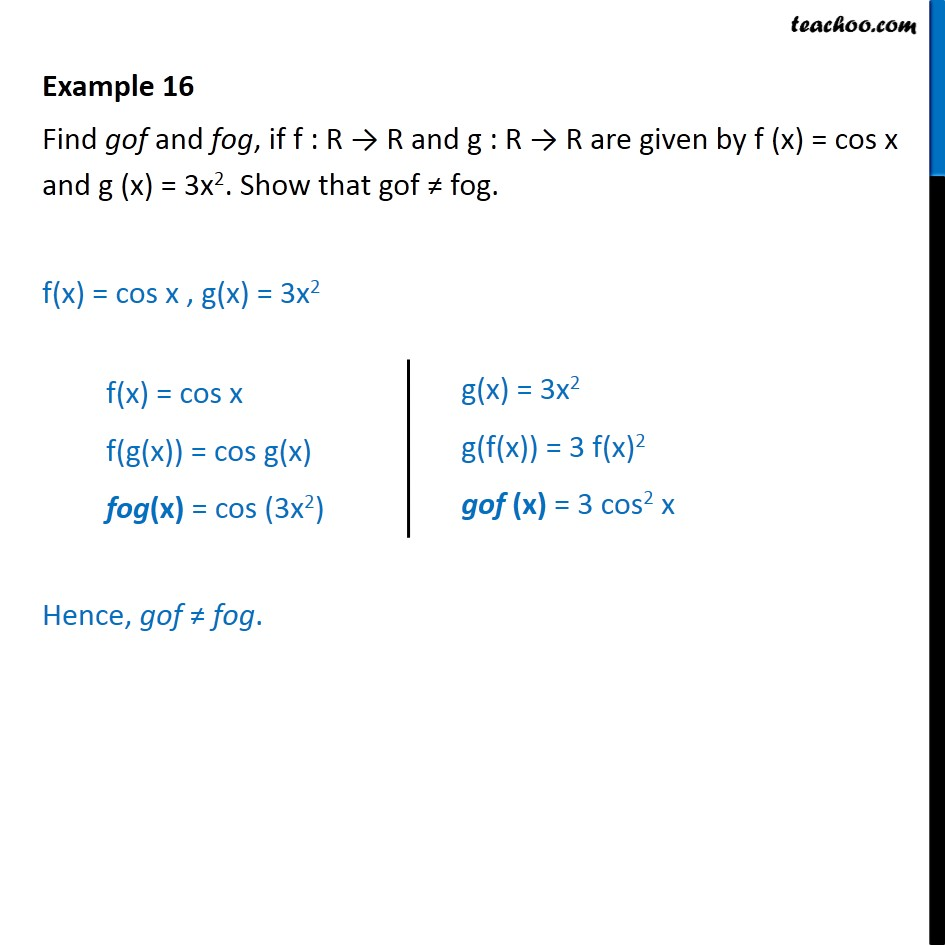Composite functions

Chapter 1 Class 12 Relation and Functions
Concept wiseLearn in your speed, with individual attention - Teachoo Maths 1-on-1 Class

### Transcript

Example 16 - Chapter 1 Class 12 Relation and Functions - NCERT Find gof and fog, if f : R → R and g : R → R are given by f (x) = cos x and g (x) = 3x^2. Show that gof ≠ fog. f(x) = cos x , g(x) = 3x^2 fog f(x) = cos x f(g(x)) = cos g(x) fog = cos 3x^2 gof g(x) = 3x^2 g(f(x)) = 3 f(x)^2 gof = 3 cos^2 x Hence, gof ≠ fog.